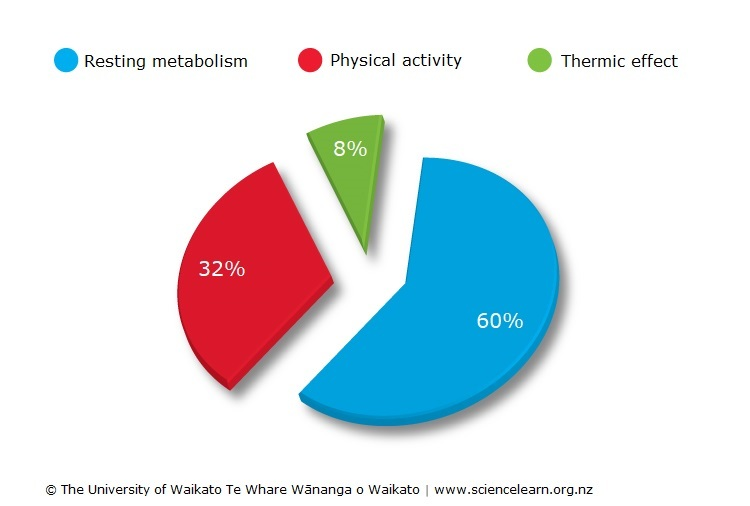d How much is 60% of 50 is greater than 40% of 30 ?

# Basic Math MCQ 7 (One number percent greater than percent of other number)

MCQ7: How much 60% of 50 is greater than 40% of 30?

a) 18                  b) 13                 c) 15                d) 20

Solution:

Here we first calculate 60% 0f 50 and then we calculate 40% of 30. The subtracting second answer from the first answer will be the result !60% 0f 50 ​$$=\frac{60}{100}\times 50=30$$

40% of 30 ​$$=\frac{40}{100}\times30=12$$Copyright secured by Digiprove © 2020